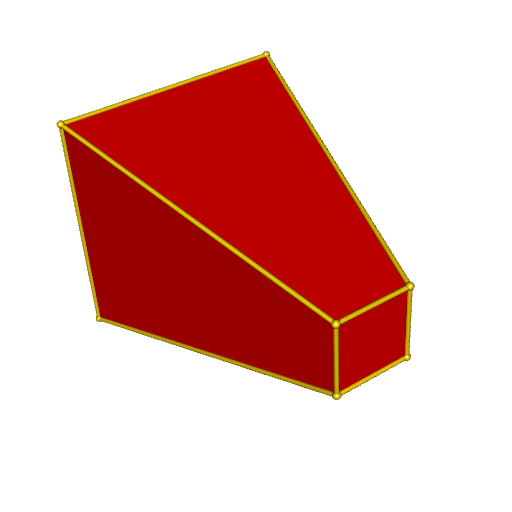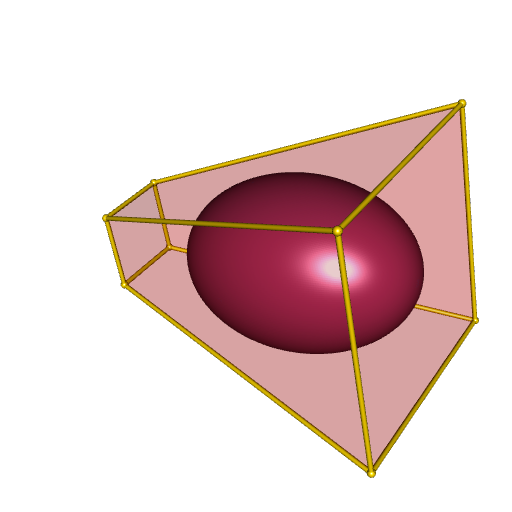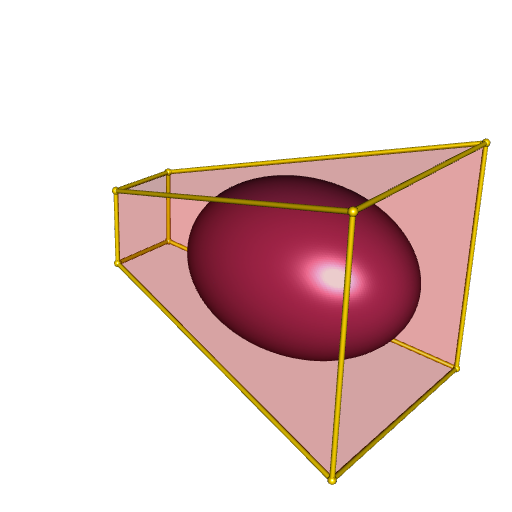# Maximum volume inscribed ellipsoid

Posted on May 20, 2023 by Stéphane Laurent
Tags: R, geometry, maths, rgl, misc3d

I’ve just discovered the excellent book Convex Optimization written by Stephen Boyd and Lieven Vandenberghe.

It gives methods for many interesting geometric problems. In this blog post I will construct the maximum volume ellipsoid inscribed in a convex polyhedron.

Let’s take the vertices of a convex polyhedron:

vertices <- rbind(
c( 0,  0,  0),
c( 0,  1,  0),
c( 1,  1,  0),
c( 1,  0,  0),
c(-1, -1,  4),
c(-1,  2,  4),
c( 2,  2,  4),
c( 2, -1,  4)
)

Let’s see how it looks like:

library(cxhull)
library(rgl)
polyhedron <- cxhull(vertices, triangulate = TRUE)
open3d(windowRect = 50 + c(0, 0, 512, 512))
plotConvexHull3d(polyhedron, facesColor = "red")The goal is to inscribe an ellipsoid in this polyhedron with the maximum volume.

We first need to represent the polyhedron by a set of linear inequalities. To do so, we use the rcdd package.

library(rcdd)
V <- makeV(vertices)     # V-representation
H <- scdd(V)[["output"]] # H-representation
A <- - H[, -c(1L, 2L)]   # matrix A
b <- H[, 2L]             # column vector b

Now we have a matrix $$A$$ and a column vector $$b$$ such that our polyhedron is defined by $$Ax \leqslant b$$.

Then we’re ready to define the optimization problem, with the CVXR package. See the book for the details

library(CVXR)
Bvar <- Variable(3, 3, symmetric = TRUE) # a symmetric 3x3 matrix
dvar <- Variable(3)                      # a length three vector
objective <- Minimize(-log_det(Bvar))    # objective
# now we define the constraints
constraints <- list()
for(i in 1L:nrow(A)) {
constraints <- append(
constraints, list(norm2(Bvar %*% A[i,]) + sum(A[i,]*dvar) <= b[i])
)
}

It remains to solve this problem.

program <- Problem(objective, constraints)
solution <- solve(program, solver = "SCS")
# extract the solutions
B <- solution$getValue(Bvar) d <- c(solution$getValue(dvar))

The matrix $$B$$ is the shape matrix of the ellipsoid and the vector $$d$$ is its center. That means that the ellipsoid is the set of vector $$Bu + d$$ for $$u$$ in the unit ball. This is a parameterization of the ellipsoid. We can plot it with the help of spherical coordinates.

library(misc3d) # to use parametric3d
h <- function(θ, ϕ) {
x <- cos(θ) * sin(ϕ)
y <- sin(θ) * sin(ϕ)
z <- cos(ϕ)
u <- c(x, y, z)
B %*% u + d
}
fx <- Vectorize(function(u, v) {h(u, v)[1L]})
fy <- Vectorize(function(u, v) {h(u, v)[2L]})
fz <- Vectorize(function(u, v) {h(u, v)[3L]})
# plot
open3d(windowRect = 50 + c(0, 0, 512, 512))
plotConvexHull3d(polyhedron, facesColor = "red", alpha = 0.2)
parametric3d(
fx, fy, fz, umin = 0, umax = 2*pi, vmin = 0, vmax = pi, n = 250,
color = "maroon", add = TRUE
)Another way to plot it is to use the central matrix of the ellipsoid, which defines the ellipsoid as an isosurface. The central matrix is $$S = {(BB')^{-1}}$$, and then the ellipsoid is the set of points $$x$$ satisfying $$(x-d)' S (x-d) \leqslant 1$$.

library(misc3d)
S <- chol2inv(chol(tcrossprod(B)))
x <- seq(-1, 2, length.out = 150L)
y <- seq(-1, 2, length.out = 150L)
z <- seq(1, 4, length.out = 150L)
Grid <- as.matrix(expand.grid(x = x, y = y, z = z))
voxel <- array(
apply(Grid, 1L, function(v) c(t(v-d) %*% S %*% (v-d))),
dim = c(150L, 150L, 150L)
)
isosurface <- computeContour3d(voxel, max(voxel), 1, x = x, y = y, z = z)
# plot
open3d(windowRect = 50 + c(0, 0, 512, 512))
drawScene.rgl(makeTriangles(isosurface), color = "maroon")
plotConvexHull3d(polyhedron, facesColor = "red", alpha = 0.2)The same method can be performed in any dimension. In the latest update of my package PlaneGeometry, I implemented it for dimension 2.